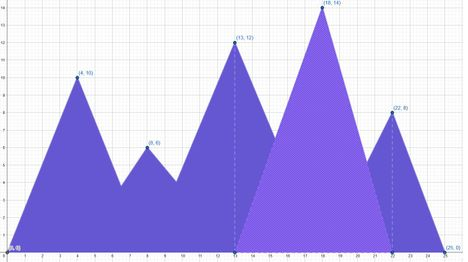# Cardboard MountainsAK_74uNational Girls' Programmi...8/10/41
Limits 1s, 512 MB

Alice has been given the responsibility of creating the background of the annual play in her school. The background consists of Mountains. One such Background-Figure 1: A Cardboard Mountain Background.

The Mountains' characteristics are —

1. Mountains are triangles whose base is on the floor.

2. A stick is placed vertically from the floor to the peak of the mountain to support it.

3. The lower right vertex of a mountain starts from the stick of the mountain to the immediate left and the lower right vertex on the stick of the mountain to the immediate right.

4. The left-most mountain's lower left vertex is on the lower left corner of the background.

5. The right-most mountain's lower right vertex is on the lower right corner of the background.

6. The width of the stick is negligible.

Note that, a mountain $m_1$ is defined left (or, right) to another mountain $m_2$ if the stick supporting $m_1$ is strictly on the left (or, right respectively) of $m_2$. Furthermore, $m_1$ is the immediate left (or, right) of $m_2$ if among all of the mountains who are on left (or, right) of $m_2$, $m_1$ is the right-most (or, left-most respectively).

The Mountains are traditionally created this way. But Alice being a problem solver has thought of an easier way to create the mountains. Alice thought of doing the following —

1. Consider the background as a 2D Cartesian plane, where the lower left corner will be the origin and the lower right corner will be $(X, 0)$, where $X$ is the length of the background.

2. Fix the coordinates of the peaks of $N$ Mountains on the Cartesian plane, then draw the Mountains respecting their characteristics, on a paper for reference.

3. Cut a single cardboard shape according to that reference.

Let’s say Alice has generated 5 mountain peaks’ co-ordinates. They are $(8, 6)$, $(22, 8)$, $(4, 10)$, $(13, 12)$ and $(18, 14)$. The length of the background is $25$ units. The final shape of the cardboard shape can be denoted by figure 1.

Alice is wondering what would be the area of the final cardboard shape for the Mountains on the background after cutting. Given the coordinates of the $N$ mountain tips, and the length of the background, $X$, can you calculate the area of the final cardboard shape?

## Input

The first line contains a single integer $T$ $(1≤T≤10^4)$, the number of test cases.

The first line of each test case contains two space-separated integers, $N$$(1 \le N \le 2 \times 10^5)$ and $X$$(2 \le X \le 10^6)$, denoting the number of peaks and the background’s length respectively.

The $i^{th}$ line of the next $N$lines contain two integers $x_i$ and $y_i$ $(0 < x_i < X, \; 1 \le y_i \le 10^6)$, the coordinate of the $i^{th}$ mountain.

The sum of $N$ all over test cases doesn’t exceed $2 \times 10^5$.

## Output

For each test case, output the area of the final cardboard shape. Your answer will be considered correct if the relative or absolute difference is less than $10^{-6}$. That means, if your answer is $A$ and jury’s answer is $B$, your answer will be considered correct if $\frac{|A-B|}{max(1,B)}<10^{-6}$.

## Sample

InputOutput
1
5 25
8 6
18 14
4 10
13 12
22 8

174.16433566Geometry

### Submit